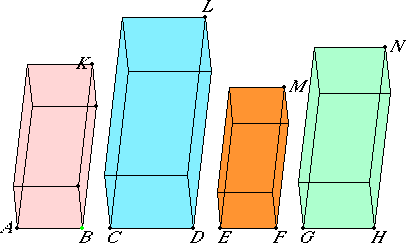# Proposition 37

If four straight lines are proportional, then parallelepipedal solids on them which are similar and similarly described are also proportional; and, if the parallelepipedal solids on them which are similar and similarly described are proportional, then the straight lines themselves are also proportional.

Let AB, CD, EF, and GH be four straight lines in proportion, so that AB is to CD as EF is to GH, and let there be described on AB, CD, EF, and GH the similar and similarly situated parallelepipedal solids KA, LC, ME, NG.

I say that KA is to LC as ME is to NG.XI.33

Since the parallelepipedal solid KA is similar to LC, therefore KA has to LC the ratio triplicate of that which AB has to CD. For the same reason ME has to NG the ratio triplicate of that which EF has to GH.

And AB is to CD as EF is to GH. Therefore AK is to LC as ME is to NG.

Next as the solid AK is to the solid LC, so let the solid ME be to the solid NG.

XI.33

I say that the straight line AB is to CD as EF is to GH. Since, again, KA has to LC the ratio triplicate of that which AB has to CD, and ME also has to NG the ratio triplicate of that which EF has to GH, and KA is to LC as ME is to NG, therefore AB is to CD as EF is to GH.

Therefore, if four straight lines are proportional, then parallelepipedal solids on them which are similar and similarly described are also proportional; and, if the parallelepipedal solids on them which are similar and similarly described are proportional, then the straight lines themselves are also proportional.

Q.E.D.

## Guide

This proposition completes the theory of volumes of parallelepipeds.

In the proof of this proposition it is assumed that two ratios are equal if and only if their triplicate ratios are equal. The required proof is long and detailed, but not difficult.